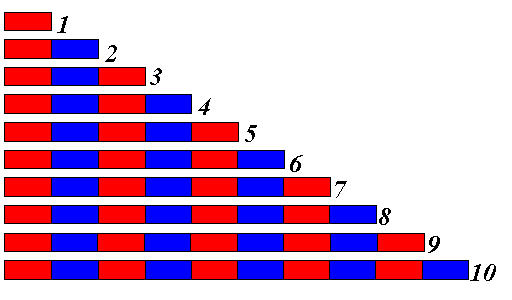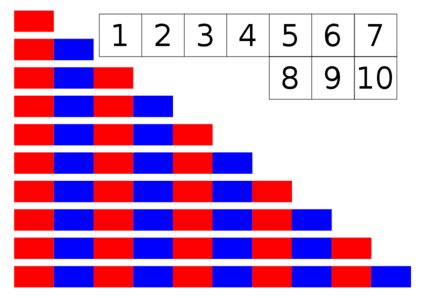# Number RodsNumber Rod Printouts

Number Rods-ages 3 ½ to 6 years old,
10 wooden rods varying in length from 10 centimeters to 1 meter. Each rod is colored in an alternating red and blue pattern. The first rod is red. The second, which is 20 centimeters divided into two 10 centimeter sections, is red and blue. The rods go on until the last rod is divided into 10 sections that are red-blue-red-blue-red-blue-red-blue-red-blue.

Exercise 1:
Have your child mix the rods on a mat. Let him or her build them into a stair like the red rods, starting from shortest to longest with the red rods even on the left side.

Exercise 2:
After this lesson is complete, count with your child using the 3 period lesson. Point to one and say, “This is one.” Point to two and say, “This is two.” Then pointing with your finger count the sections, “one, two.” Start with 2 or 3 number rods to begin with. Add number rods as your child masters the lesson. Repeat until your child really understands counting and the association of numbers to the rods.

Exercise 3:

Mix the number rods. Randomly ask your child to give you the correct rod for the number you are asking for. For example, “Give me two.” The child gives you the rod with two sections. When your child gives you the rod, have her or him count the number of sections. When your child does this successfully, go onto the 4th exercise.

Exercise 4:
Show your child a rod and ask him or her to count it and tell you the name. When your child knows all the first three rods, add more until your child can count all the rods and tell you the name.
Your child should be able to put the rods in a stair and count them as he or she does the lesson.

Exercise 5:
Have your child build the stair and do the following. Point to number rod two and say, two is one more than one. Point to number rod three and say, three is one more than two.” Add a few more each day, as your child understands the concept.

Exercise 6:
Have your child help you put the number rod stair in order. Place the corresponding number by the correct number rod.
Say to your child, when your put the number 1 by the 1st number rod, (again use the 3 period lesson) “This is the number one” By the second number rod, the number 2, and say “This is the number 2.”
"Show me the number one, show me the number two." Point to the numbers and ask your child, “What number is this.”
If your child has difficulty with this go back to previous stage. Do a few rods and numerals at a time until your child is comfortable with the lesson. Let your child do the entire lesson when ready.

Exercise 7: When your child is familiar with the numbers and the number rod quantities your can use the number rods to teach addition. Show how 3 number rod plus the 5 number rod is the same as the 8 number rod. 1 rod plus the 2 number rod is equal the number 3 rod.

Also you can show variations of addition.
For example:
1 number rod plus 4 number rod equals 5
2 number rod plus 3 number rod equals 5

Purpose: Learn the names of numbers 1-10 and visually associate the numbers with the quantity. This teaches numeral recognition, quantity and addition readiness.

Number Rods and Red Rods Extension

Similar Pages:Lesson of the Day 3

Become a Patron!